# Abstract

This paper presents analytical and experimental results on ductility of reinforced lightweight concrete beams and columns in the form of moment curvature relationships, and compares the response with that of normal reinforced concrete members. The experimental part is limited to flexural tests on beams made of lightweight concrete. The latter is obtained with natural lightweight aggregates. Concrete and steel stress-strain models in compression and tension are integrated analytically through the section in order to derive the resulting moment and axial force. Lightweight concrete beams and columns showed a more ductile behavior than normal concrete members and the analytical model reproduced the response with very good accuracy. The lightweight ductility was more pronounced in columns subjected to axial compression forces and bending.

Lightweight concrete; moment curvature; stress integration; ductility

Ductility of reinforced lightweight concrete beams and columns

Abdelhamid Charif* * Author email: acharif@ksu.edu.sa ; M. Jamal Shannag; Saleh Dghaither

King Saud University

ABSTRACT

This paper presents analytical and experimental results on ductility of reinforced lightweight concrete beams and columns in the form of moment curvature relationships, and compares the response with that of normal reinforced concrete members. The experimental part is limited to flexural tests on beams made of lightweight concrete. The latter is obtained with natural lightweight aggregates. Concrete and steel stress-strain models in compression and tension are integrated analytically through the section in order to derive the resulting moment and axial force. Lightweight concrete beams and columns showed a more ductile behavior than normal concrete members and the analytical model reproduced the response with very good accuracy. The lightweight ductility was more pronounced in columns subjected to axial compression forces and bending.

Keywords: Lightweight concrete, moment curvature, stress integration, ductility

1 INTRODUCTION

Lightweight concrete is no longer considered a special material. It is included in many codes of practice, such as the American Concrete Institute ACI (1987), as normal concrete but with a lower density and increased deformability. The LWC material properties and mechanics have long been identified and still continue to attract interest as shown by the Works of Hon Zhi (2007), Koh et al. (2008) and Muyasser et al. (20011). Many structural and bridge applications have been reported by authors such as Russel (2007) and Waldron et al. (2005). High strength has also been achieved by Katkhuda et al. (2009), Yasar et al. (2004) and others. LWC offers undeniable isolation advantages but the reduction in the overall cost generated by the lower dead loads is often overwhelmed by the higher production cost, especially with factory produced expanded clay lightweight aggregates. LWC becomes however more challenging when using natural volcanic rocks reserves to produce lightweight aggregates. On the other hand, even if the reduced stiffness of LWC concrete requires a tighter deflection control, its higher ultimate strain confers a major advantage to LWC in the form of improved ductility and better energy absorption capacity.

2 ANALYTICAL MOMENT-CURVATURE RELATIONSHIP

An analytical integration method of stress resultants over an arbitrary shaped section was formulated and used to compute the axial force and moment. This method, dealing with biaxial bending combined with an axial force, is described in detail in the Works of Zupan, Saje (2005) and Charif et al. (2012). It performs better than the various numerical integration techniques such as those described by Sfakianakis (1999), Bonet et al. (2006) and Charalampakis, Koumousis (2008). The main steps are summarized here. Using the direct sign convention shown in Figure 1, the normal stress resultants for a beam-column member, subjected to an axial force and biaxial bending, are given by:3 STRESS RESULTANTS FOR DISCRETE BAR REINFORCEMENT

Reinforcing bars are lumped at their centroid points with coordinates ybi and zbi. Their contribution is easily determined from the corresponding area and stress. To account for the displaced embedding concrete, an equivalent stress is subtracted.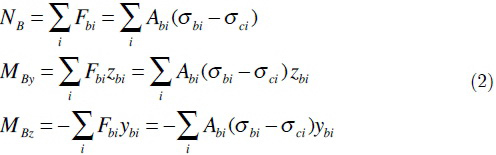Bar stress (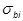) and concrete stress () at the same point i are easily computed using corresponding stress-strain models. The capacity of a section with discrete reinforcement is obtained by combining equations (1) and (2). All other forms of non-discrete reinforcement use equations (1) by summing integrations over all the components of the section.

The axial force in (1) and (2) is independent of y-z axis system whereas bending moments depend on the origin and orientations of these axes. It is common to express moments about the cross section centroid. This point is thus considered as the origin. With the assumption of plane sections and strain compatibility (perfect bond between various components), the total normal strain is a combination of axial force and bending moment effects:is the centroidal normal strain, whereasandare curvatures about respective axes.

For dissymmetric sections, bending about one axis causes curvatures about both axes and bending angle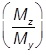is in general different from neutral axis angle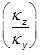.

4 EXPRESSIONS OF INTEGRALS IN LOCAL NEUTRAL COORDINATES

Normal strain (3) and the resulting normal stress are functions of both y and z coordinates. There exists a system of axes (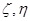) as shown in Figure 1 where normal strain and stress depend on coordinate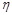only (-axis parallel to the neutral axis).Integral relations (1) can be equally expressed with respect to coordinates (), and then by virtue of relation (4) with respect to coordinates (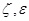). We obtain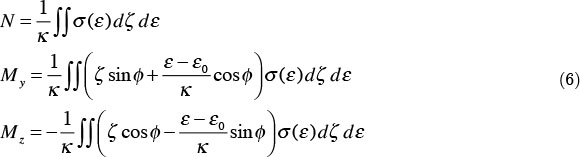5 BOUNDARY INTEGRATION OF STRESS RESULTANTS

Green's theorem is a very useful tool as it reduces the problem dimension by replacing area integrals by path integrals along the border. The resulting boundary integration method offers many advantages and is very convenient for complex sections as it can elegantly handle various parts including voids. Figure 2 shows a composite section with three parts. The inner zone is either a void or a different material part. Figure 2b shows the appropriate vertex numbering (12 points) for the main part which eliminates the inner one, thanks to direction sign dependence of line integrals. If the inner part is not a void, it is dealt with similarly by a vertex numbering in the counterclockwise direction. The external skin reinforcement is also treated separately in the same way.

Figure 3 shows vertex numbering for the original section as well as identification of vertices for various parts. It is supposed in this case that both compression and tension models have two equations each as shown. The various parts are defined by lines parallel to the neutral axis, resulting in sub-polygons. The vertices for each part are shown in Table 1.

Applying Green transformations to equations (6) leads to:These integrations (7) are performed along the border lines of the section.

For a polygonal section, vertex points are expressed in (y , z) coordinates and then transformed to () coordinates. Each polygon side is identified by two successive vertices (v) and (v+1) with a linear relation between their coordinates:nv is the total number of vertices and point (nv +1) refers to the first vertex in order to complete the closed boundary integral. Substitution and arrangement lead to:With:The three different integrals in the previous relations are:6 ANALYTICAL BORDER INTEGRATION OF POLYNOMIAL MODELS

Nonlinear stress-strain models are usually expressed by one or two equations and the integrals (11) cannot be derived analytically in all cases. They are straightforward for polynomial models. For a polynomial stress-strain model of order k:The three integrals (11) become:Relations (9) and (13) deliver closed-form solutions of normal stress resultants. It can be seen from (13) that all boundary lines parallel to the neutral axishave no contributions to force and moment resultants. This in fact is true by virtue of (11) and (9) whether the model is polynomial or not.

This formulation cannot however be used for non-integrable material models. An alternative to using numerical integration, is replacing the original model by an appropriate polynomial fit and then use the present method. Charif et al. (2012) proved that this was more efficient than performing numerical integration on original models.

Apart from the moment-curvature relationship for a given constant axial force, the preceding formulation can also be used to determine N-My-Mz interaction curves and surfaces, and the stress state for a given loading.

7 EXPERIMENTAL VALIDATION ON BEAM FLEXURE

7.1 Introduction

The present work is part of a strategic and ambitious project to promote LWC in Saudi Arabia using large reserves of natural basalt tuffs (scoria) and volcanic rocks available in some northwestern parts of the country, as reported by the Saudi Ministry of Petroleum (1998). Figure 4 shows some volcanic rock samples and the aggregates obtained after crushing and sieving. The various properties of these aggregates and the mix design techniques used are described by Shannag et al. (2013). With a weight ranging from 1750 to 1950 kN/m3, compression strengths from 18.0 to 45.0 MPa, were obtained. Two concrete grades (25 and 35 MPa) are used in this work. Their stress-strain relationships, measured under displacement controlled loading, are shown in Figure 5.

7.2 Experimental flexural beam tests

Full scale beams were tested under third point loading until failure for both concrete grades. The under reinforced beams were provided with sufficient stirrups to prevent shear failure (Figure 6). The bottom tensile steel consisted of three bars of 16 or 20 mm diameter, corresponding to 0.9% and 1.5% steel ratios. The beams were loaded up to failure and the curvature was tracked through material strains using electrical gauges and transducers.

8 MATERIAL MODELING AND MOMENT-CURVATURE RESPONSE FOR BEAMS

8.1 Material modeling

An integrated modeling strategy is used. The LWC material stress-strain models are first approached by polynomials using an efficient constrained least square method. The resulting polynomial model is then analytically integrated to compute the stress resultants. The parameters of the equivalent rectangular stress block, having the same area and centroid as the original stress-strain curve, are also delivered. Polynomial modeling for the two lightweight concrete grades used is shown in Figure 7. In each case two polynomials of rank 4 or 5 are used. The rank is adjusted until the desired accuracy is achieved.

Concrete tension model and steel bar model are also analytically integrated. Concrete tensile stress-strain model was not directly recorded but it was based on the measured modulus of rupture and assuming a parabolic variation with a quadratic decay (tension stiffening) as shown in Figure 7. The 16 and 20 mm reinforcing steel bars recorded an average yield strength of 425 MPa and exhibited strain hardening behavior up to an ultimate stress of 660 MPa (Figure 8). The nonlinear strain hardening was modeled using Rasmussen (2001) modified equation of Ramberg-Osgood.

8.2 Moment-Curvature algorithm

The steps to compute moment curvature up to failure are:

1. Determine the initial section stress state under the constant axial force (if any).

2. Increase the top concrete strain using appropriate steps and for each level:

● Update tension steel strain to satisfy force equilibrium

● Compute corresponding moment and curvature

The process is usually stopped when a failure criterion is reached. In this work the section strength is computed at any straining level, even beyond the material ultimate strains, and the algorithm is stopped only if there is a major strength drop. This allows load transfer between the various section components and is particularly convenient for analysis of complex composite sections with many parts and different material properties. Limited local failures as well as the spread of crushing inside the concrete cover can thus be captured. Some significant points, such as the cracking and yielding moments, may be skipped by this incremental procedure. They are therefore determined separately and then added to the recorded points.

Figures 9 and 10 show the measured and predicted moment-curvature responses for both LWC grades and the two steel ratios used. These curves show a very good concordance at all load levels and confirm the reduced ductility with higher reinforcement ratios. The stiffness reduction after cracking is more important with a lower steel ratio. The three major stages (uncracked, cracked service, cracked ultimate) are clearly illustrated. The stiffer uncracked stage and the hardening ultimate stage reflect the effect of both tensile concrete as well as steel strain hardening. Figure 11 shows the deviation between the measured and predicted moment curvatures when neglecting both concrete tension and steel hardening.

8.3 Moment-Curvature and ductility of normal and lightweight concrete beams

The previous LWC beam responses are now compared to similar normal concrete (NC) beams. A similar strength normal concrete is used with 0.004 ultimate strain and Thorenfeldt et al. model (1987) which is valid for normal and high strength concrete. This model uses a single equation but with different parameters for the ascending and descending branches:This model cannot be integrated analytically and the stress resultants must therefore be numerically integrated. Alternatively the original model may be approximated to any desired accuracy by polynomials which can then be integrated analytically. Figure 12 shows the polynomial fitting for the two parts. Using fourth rank polynomials reproduces the original equations almost to perfection.

Figure 13 shows the moment curvature responses of NC and LWC beams for both steel ratios. The strength capacity is similar but the NC beams exhibit a better initial stiffness whereas the LWC beams develop more ductility.

9 MOMENT-CURVATURE AND DUCTILITY OF COLUMNS

9.1 Introduction

Beams are usually designed as under reinforced and tension controlled sections with a sufficient ductility. Concrete ductility is more needed in columns, which are often subjected to axial compression forces reducing their energy absorption capacity. A validation of the theoretical model developed is first presented using experimental results from literature with a confined concrete model, and then a parametric investigation is carried out using the previous normal and lightweight concrete models.

9.2 Validation

Experimental data on moment-curvature of reinforced columns is rather scarce. Testing of columns under increasing lateral load up to failure, while maintaining a constant axial force, is difficult. Tests using an eccentric axial force have also been reported but these are different since both axial foce and moment vary at the same time. The present validation uses results reported by Sheikh et al. (1990). They tested several square (305 x 305 mm) columns under various levels of axial force and different configurations of transverse steel ties, in order to study their lateral confinement effect. In a follow up paper, Sheikh et al. (1992), the same authors reported the inability of most confined concrete models to reproduce their experimental results. The recent model proposed by Reddiar (2009) was used because of its integrable form (Figure 14), its simplicity, and its validity for both normal and high strength concrete.

The confined model is described by an ascending power equation followed by two linear descending parts :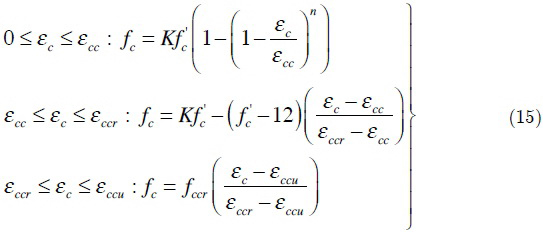The model is completely defined by the confining strength parameter K and the values of the crushing and ultímate strains (εccr and εccu). This offers more versatility to the model as it can easily be adapted to various confining configurations for circular or rectangular columns. The other model parameters are defined as follows:The linear parts are integrable as first rank polynomials. The power function of the first part is not polynomial but can be integrated analytically. The three corresponding integrals (11) are :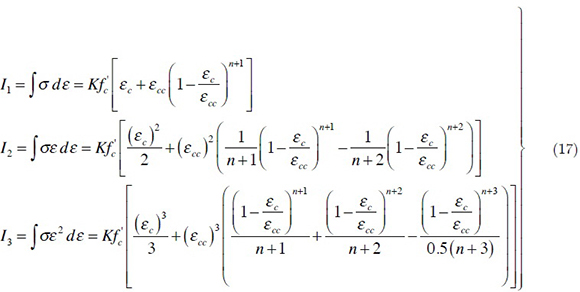This model was implemented using the previously described strategy in order to predict the moment-curvature response of two columns E2 and E13 tested by Sheikh et al. (1990). The columns have the same longitudinal steel (eight 19-mm bars) and same 12.7-mm lateral ties spaced at 114 mm. They differed by the concrete strength (31.4 and 27.3 MPa) and by the axial force value (1782 and 1879 kN). The column section and material models are shown in Figure 15, with a confinement parameter value of 1.14.

Figure 16 shows experimental and predicted moment-curvature relations for both columns E2 and E13. The analytical integration method proves itself, once again, very efficient in predicting with very good accuracy the response of RC columns, provided the adequate material models are used.

9.3 Column ductility investigation

A parametric investigation is now performed using previous models, used in beams, for normal and lightweight concrete. A square column 400x400 mm with eight 16 or 30 mm bars is now studied using grade 25 normal and lightweight concretes. Figures 17 and 18 show the column moment-curvature response at various axial force levels for both normal and lightweight concrete with 1.57% and 3.5% steel reinforcement respectively. Lightweight concrete ductility is better than that of normal concrete in all cases at any level of the axial force including negative tension values. The advantage of LWC is more pronounced with higher steel ratio and increased compression forces (Figures 17 and 18).

10 CAPTURE OF LOCAL AND PARTIAL FAILURE

As mentioned previously, the strategy used in this work allows tracking any possible response after reaching any material ultimate strain. Figures 19 and 20 show the ability of the present analytical integration method to track the complex load transfer mechanism after local failure. Figure 19 shows the capture of the spread of concrete crushing inside the cover (from point B to point D) for the previous 400x400 mm LWC column. Figure 20 shows the progressive steel rupture in a multi-layer beam. It must be pointed out that Charif et al. (2012) showed that numerical integration methods failed to track the spread of crushing inside the concrete cover.

11 CONCLUSIONS

Experimental and theoretical moment-curvature responses of normal and lightweight reinforced concrete beams and columns were presented. Lightweight concrete members developed more ductile behavior than their normal concrete counterparts, and this enhanced ductility was more pronounced in columns subjected to axial compression forces. The theoretical model, based on analytical integration of stress resultants through the cross-section, predicted the moment-curvature response with very good accuracy. It also predicted with very good satisfation the moment-curvature response of reinforced concrete columns with lateral steel providing confinement effect. This method, which is also valid for the analysis of biaxial bending and interaction surfaces, is not only more economical than numerical integration techniques, but allows a better capture of the sensitive ultimate response. The method was more appealing when combined with material modeling using an efficient constrained least square method for polynomial fitting of experimental stress-strain results. It was also further successfully extended to existing non-integrable material models using the same polynomial approach.

Acknowledgement The authors wish to acknowledge the financial support provided by National Plan for Science, and Technology, (NPST), at King Saud University, Project Number: 10-ADV-1190-02. The authors are grateful to the engineers and the technicians at the concrete and structural laboratories for their assistance during the execution of the experimental program.

• ACI 213R-87 (1987). Guide for Structural Lightweight Aggregate Concrete. American Concrete Institute, Detroit, Michigan.
• Hong Zhi C. (2007). Mechanical properties of lightweight aggregate concrete - Effect of lightweight aggregates on concrete. PhD thesis, Hong Kong University, 270p.
• Koh C. G., Teng M. Q., and Wee T. H. (2008). A Plastic-Damage Model for Lightweight Concrete and Normal Weight Concrete. International Journal of Concrete Structures and Materials, Vol.2, No.2, pp. 123~136, December 2008
• Muyasser M. J., Daham H.A., Saad M. R. (2011). Flexural behavior of lightweight concrete beams. European Journal of Scientific Research, Vol.58 No.4, pp.582-592
• Waldron, C. J., et al. (2005). Demonstration of Use of High-Performance Lightweight Concrete in Bridge Superstructure in Virginia", Journal of performance of Constructed Facilities, Vol. 19, No. 2, May, pp. 146-154.
• Russell, Henry (2007). Current Provisions and Needed Research for Lightweight Concrete in Highway Bridges. Publication No. FHWA-HRT-07-051, US Department of Transportation.
• H. Katkhuda, B. Hanayneh and N. Shatarat (2009). Influence of silica fume on high strength of lightweight concrete. World Academy of Science, Engineering and Technology, 58
• Yasar E., Atis C.D., Kilic A. (2004). High strength lightweight concrete made with ternary mixtures of cement, fly ash, silica fume, and scoria as aggregates. Turkish Journal of Engineering, Environment and Science, No 28, pp.95-100
• Charif A., Shannag M.J., Dghaither S. (2012). Analytical integration of material stress fields for section analysis and structure response. Séminaire International Risques et Génie Civil, Université de Batna 26/27 Novembre, 21p
• Zupan D., Saje M. (2005). Analytical integration of stress field and tangent material moduli over concrete cross-sections. Computers and Structures, (85), pp.2368-80
• Sfakianakis M.G. (1999). Biaxial bending with axial force of reinforced, composite and repaired concrete sections of arbitrary shape by fiber model and computer graphics. Journal of Structural Engineering, 125(6):672 - 83.
• Bonet J.L. , Barros M.H., Romero M.L. (2006). Comparative study of analytical and numerical algorithms for designing reinforced concrete sections under biaxial bending. Computers and Structures, Vol.84, pp.2184-2193
• Charalampakis A.E., Koumousis V.K. (2008). Ultimate strength analysis of composite sections under biaxial bending and axial load. Advances in Engineering Software 39, 923-936
• Ministry of Petroleum and Mineral Resources, Directorate General of Mineral Resources (1998). Atlas of Industrial Minerals, Basalt in Saudi Arabia. Kingdom of Saudi Arabia, p-24, 1419 Hijri.
• Shannag M.J., Charif A., Dghaither S. (2013). Developing structural lightweight concrete using volcanic scoria available in Saudi Arabia. Accepted for publication in Arabian Journal of Science and Engineering, 20p.
• Thorenfeldt E., Tomaszewicz A., Jensen J.J. (1987). Mechanical properties of high strength concrete and application to design. Proceedings of the symposium: Utilization of high strength concrete, Stavanger, Norway, June, pp.149-159
• Sheikh S.A.,Yeh C.C. (1990) Tied concrete columns under axial load and flexure. Journal of Structural Engineering, ASCE, Vol. 116, No. 10, pp.2780-2800
• Sheikh S.A.,Yeh C.C. (1992) Analytical moment-curvature relations for tied concrete columns. Journal of Structural Engineering, ASCE, Vol. 118, No. 2, pp.529-544
• Rasmussen K.J.R. (2001) Full range stress-strain curves for stainless steel alloys Research report R811, Department of Civil Engineering, University of Sydney, 44 pages
• Reddiar M.K.M (2009) Stress-strain model of unconfined and confined concrete and stress-block parameters. Msc Thesis, Pondicherry Engineering College, India
• *
Author email:

# Publication Dates

• Publication in this collection
13 Mar 2014
• Date of issue
Dec 2014
Associação Brasileira de Ciências Mecânicas Av. Rio Branco, 124/14º andar, 20040-001 Rio de Janeiro RJ Brasil, Tel.: (55 21) 2221 0438 - Rio de Janeiro - RJ - Brazil
E-mail: abcm@abcm.org.br